#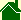The Strong Force

The "Strong Force" has long been considered one of the fundamental forces in physics. The Ball-of-Light Particle Model describes this force as a combination of the what may really be the fundamental forces: electric, magnetic, and gravitational.

### The Electric Field

It is well known that two electric charges of the same type repel each other, while two electric charges of opposite type attract each other. Using the Ball-of-Light Particle Model to visually represent these fields, one particle could be visualized as having electric fields sweeping from left to right.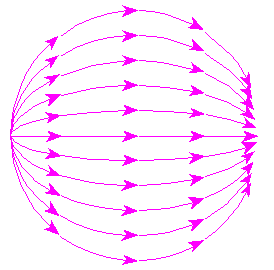A particle of opposite electrical charge could be visualized as: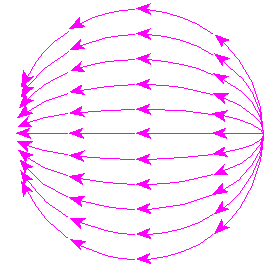If these two waves are superimposed, they would attract each with great force and become concentric waves sweeping in opposite directions.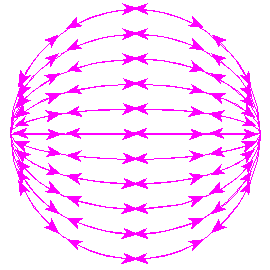It would be difficult to separate such fields due to their natural attraction.

### The Magnetic Field

It is well known that magnetic fields of similar polarity repel, and opposite polarity attract. Using the Ball-of-Light Particle Model to visually represent these fields, one particle could be visualized as having magnetic fields as if induced from electric fields sweeping from left to right.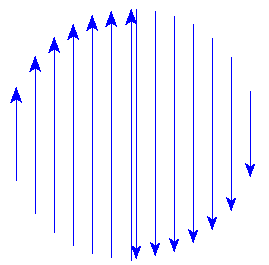A particle of opposite magnetic polarity could be visualized as: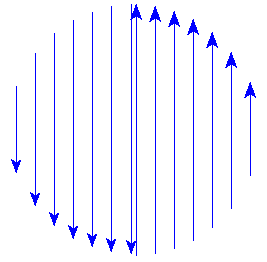If these two waves are superimposed, they would attract each with great force and become concentric waves sweeping in opposite directions.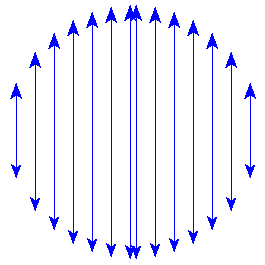It would be difficult to separate such fields due to their natural attraction.

### The Gravitational Field

A particles gravitational field points directly towards the center of the particle. If one such particles field were combined with another such particles field, the combination, as visualized using the Ball-of-Light Particle Model would appear the same when represented with vectors.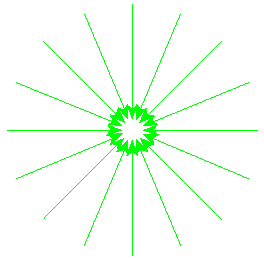+=It would be difficult to separate such fields due to their natural attraction.

### The "Strong" Force

According to the Ball-of-Light Particle Model each of these representations of Electric, Magnetic, and Gravitational fields would occur in all particles -- with differing levels of harmony. (A good example of a very harmonic particle is the neutrino.) If all of these forces are present at the same time, they would combine creating a very strong force. This very strong combination of electric, magnetic, and gravitational fields is -- according to the Ball-of-Light Particle Model -- equivalent to the traditional "Strong" force in physics. Since this force can be described by these three other forces, it is not considered fundamental. Instead, it is considered as a derived force.

The Ball-of-Light Particle Model would visualize such a superposition of forces as:Imagine trying to separate the two particles (or waves) from each other when all of the fields are attractive.

Over the years, I have heard many descriptions of the Strong force. Personally, I believe this is a very simple and intuitive description. What do you think?

Kind of makes you want to brush up on sines, cosines, and tangents doesn't it? I can see triangles of Pythagreas everywhere! I mean it! Isn't this a thing of beauty? The symmetry is perfect!